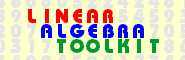© 2000−2019  P. Bogacki Calculating the determinant using row operations v. 1.25

 PROBLEM TEMPLATE

Calculate the determinant of the given n x n matrix A.

 SPECIFY MATRIX DIMENSIONS

Please select the size of the square matrix from the popup menu, click on the "Submit" button.

• Number of rows (equal to number of columns):   n = .